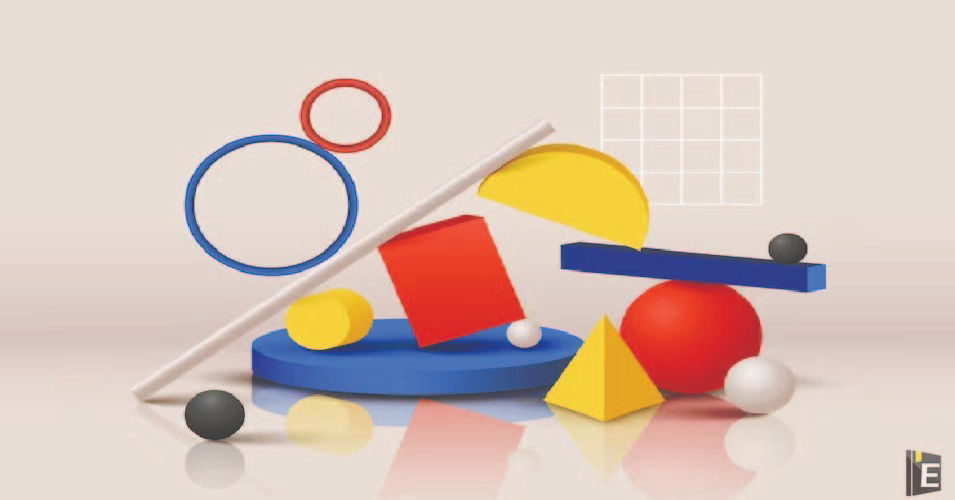• Save

# Math Questions Answer for Competitive Exams

NEWMathematical problem-solving is an integral part of various academic disciplines and practical applications. Whether it's in the fields of physics, engineering, finance, or computer science, the ability to tackle mathematical questions is a valuable skill. To begin, we examine the importance of understanding the problem statement thoroughly. We emphasize the significance of identifying key information, variables, and relationships between different elements. By breaking down the problem into manageable parts and formulating a clear objective, we set the stage for a successful solution.

In this article Math Questions and Answers, we are providing the most and latest important questions related to aptitude and general math. We delve into the art of answering math questions and exploring strategies and techniques that can help both students and professionals excel in problem-solving.

Also, Read Latest Current Affairs Questions 2023: Current Affairs Today

"Put your knowledge to the test and excel with our General Knowledge Mock Test and Current Affairs Mock Test!"

## Math Questions Answer for Competitive Exams

Q :

At a clearance sale, a shopkeeper gives 45% discount. If a customer paid ₹330 during the sale, then what is the marked price of that shirt?

(A) ₹550

(B) ₹600

(C) ₹500

(D) ₹650

Q :

If 20% of (A + B) = 30% of (A − B), then what percentage of B is equal to A?

(A) 400%

(B) 300%

(C) 500%

(D) 100%

Q :

Find the coordinates of the points where the graph 57x-19y=399 cuts the coordinate axes.

(A) x-axis at(-7,0) and y-axis at (0,-21)

(B) x-axis at(-7,0) and y-axis at (0,21)

(C) x-axis at (7,0) and y-axis at (0,-21)

(D) x-axis at (7,0) and y-axis at (0,21)

Q :

Rajnish borrowed ₹1,500 from a bank and repaid the entire amount with interest in two equal annual instalments, the first instalment being paid a year after Rajnish borrowed from the bank. If the rate of interest was 40% per annum, compounded annually, then what was the value (in ₹) of each instalment paid by Rajnish?

(A) 1125

(B) 1470

(C) 1225

(D) 1350

Q :

A sum triple itself in 9 years at simple interest. Find the rate of interest per annum is.

(A) $$38{2\over 9} \%$$

(B) $$33{1\over 3} \%$$

(C) $$27{1\over 9} \%$$

(D) $$22{2\over 9} \%$$

Q :

A starts a business with ₹75,000 and B joins the business 5 months later with an investment of ₹80,000. After 1 year, they earn a profit of ₹4,08,800. Find the share of A and B (in ₹).

(A) 2,52,000 and 1,56,800

(B) 2,50,000 and 1,58,800

(C) 2,48,000 and 1,60,800

(D) 2,49,500 and 1,59,300

Q :

(sinθ+cosecθ)2+(cos θ+ sec θ)2=?

(A) 5 + tan2 θ + cot2 θ

(B) 7 + tan2 θ - cot2 θ

(C) 7 + tan2 θ + cot2 θ

(D) 5 + tan2 θ - cot2 θ

Q :

The value of $${0.7×0.7×0.7+0.3×0.3×0.3}\over {0.7×0.7-0.7×0.3+0.3×0.3}$$ is

(A) 2

(B) 1

(C) 3

(D) -1

Q :

if one of the interior angles of a regular polygon is $$15\over 16$$ times of one of the interior angles of a regular decagon, then the number of diagonal of the polygon is.

(A) 20

(B) 14

(C) 2

(D) 35

Q :

Which of the following numbers is divisible by 11?

(A) 5214341

(B) 5648741

(C) 6598321

(D) 2378965